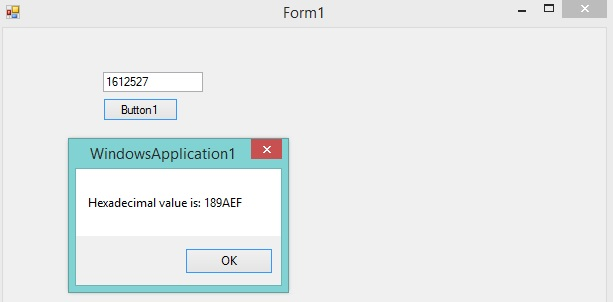Code Snippets VB.Net

# VB.Net - Convert Integer (Decimal) to Hexadecimal String.

IncludeHelp VB.Net 13 OCT 2016

In this VB.Net code we will learn how to convert a decimal integer value to its equivalent hexadecimal string?

In this example we created a sample form with two components textbox and button, conversion operation will be performed on button click event and value will be entered in textbox.

To convert decimal integer value to Hexadecimal string we used Hex() Method which will convert given Integer Value to Hexadecimal String.

Here is the syntax

`Hex(integer_value)`

## VB.Net Code - Convert Decimal (Integer) to Hexadecimal String

```Public Class Form1

Private Sub Button1_Click(ByVal sender As System.Object, ByVal e As System.EventArgs) Handles Button1.Click
'get the value from text box - value should be in hex string

Dim decValue As Integer = 0
Dim hexString As String = Nothing

decValue = Int(TextBox1.Text.Trim)

hexString = Hex(decValue)

MsgBox("Hexadecimal value is: " & hexString)

End Sub
End Class
```

### Output